# Exact solution of the Percus Yevick integral equation for hard spheres

The exact solution for the Percus Yevick integral equation for the hard sphere model was derived by M. S. Wertheim in 1963  (see also ), and for mixtures by Joel Lebowitz in 1964 .

The direct correlation function is given by (Eq. 6 of  )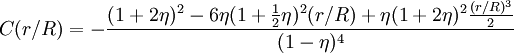$C(r/R) = - \frac{(1+2\eta)^2 - 6\eta(1+ \frac{1}{2} \eta)^2(r/R) + \eta(1+2\eta)^2\frac{(r/R)^3}{2}}{(1-\eta)^4}$

where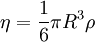$\eta = \frac{1}{6} \pi R^3 \rho$

and$R$ is the hard sphere diameter. The equation of state is given by (Eq. 7 of )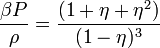$\frac{\beta P}{\rho} = \frac{(1+\eta+\eta^2)}{(1-\eta)^3}$

where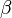$\beta$ is the inverse temperature. Everett Thiele also studied this system , resulting in (Eq. 23)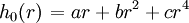$\left.h_0(r)\right. = ar+ br^2 + cr^4$

where (Eq. 24)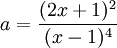$a = \frac{(2x+1)^2}{(x-1)^4}$

and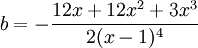$b= - \frac{12x + 12x^2 + 3x^3}{2(x-1)^4}$

and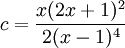$c= \frac{x(2x+1)^2}{2(x-1)^4}$

and where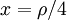$x=\rho/4$.

The pressure via the pressure route (Eq.s 32 and 33) is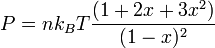$P=nk_BT\frac{(1+2x+3x^2)}{(1-x)^2}$

and the compressibility route is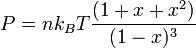$P=nk_BT\frac{(1+x+x^2)}{(1-x)^3}$